# Obey validity limits of data-driven models through topological data analysis and one-class classification

## Abstract

Data-driven models are becoming increasingly popular in engineering, on their own or in combination with mechanistic models. Commonly, the trained models are subsequently used in model-based optimization of design and/or operation of processes. Thus, it is critical to ensure that data-driven models are not evaluated outside their validity domain during process optimization. We propose a method to learn this validity domain and encode it as constraints in process optimization. We first perform a topological data analysis using persistent homology identifying potential holes or separated clusters in the training data. In case clusters or holes are identified, we train a one-class classifier, i.e., a one-class support vector machine, on the training data domain and encode it as constraints in the subsequent process optimization. Otherwise, we construct the convex hull of the data and encode it as constraints. We finally perform deterministic global process optimization with the data-driven models subject to their respective validity constraints. To ensure computational tractability, we develop a reduced-space formulation for trained one-class support vector machines and show that our formulation outperforms common full-space formulations by a factor of over 3000, making it a viable tool for engineering applications. The method is ready-to-use and available open-source as part of our MeLOn toolbox (https://git.rwth-aachen.de/avt.svt/public/MeLOn).

## Introduction

Supervised machine-learning techniques have been re-emerging as a promising avenue for data-driven modeling in various engineering disciplines (Venkatasubramanian 2019). In most applications, the overall goal is the optimal decision-making based on available data and a priori knowledge. Thus, data-driven models and mechanistic models are often combined to form hybrid models (Mogk et al. 2002; Kahrs and Marquardt 2008; Von Stosch et al. 2014; Glassey and Von Stosch 2018). Subsequently, hybrid models are frequently used in model-based optimization of design and/or operation of processes (McBride and Sundmacher 2019; Schweidtmann and Mitsos 2019).

A critical issue of data-driven models is their limited extrapolability. Unless strong assumptions are posed on the learned function, data-driven models can only be valid in regions where they have sufficiently dense coverage of training data points. We refer to this as the validity domain (Leonard et al. 1992; Courrieu 1994). Consequently, there is a need to avoid the evaluation of data-driven models outside their validity domain during optimization. Note that we refer to the validity domain of individual data-driven models throughout this work, but the concept can also be applied to hybrid models (Kahrs and Marquardt 2007).

The vast majority of previous publications use box constraints (i.e., hyperrectangles) to bound the inputs of data-driven models, i.e., each variable has independent bounds. This approach is practical when the training data is obtained from simulations based on regular grids or Latin hypercubes that are sufficiently dense. It is also advantageous for local and global optimization. However, it requires a priori known bounds and the possibility to obtain training data for any input combination. In practice, simulations can fail (Asprion 2020) and industrial process data usually does not cover hyperrectangular spaces (Asprion et al. 2019). This leads to manual selection of wrong bounds, which may cut off optimal solutions or overestimate the validity domain.

As proposed by Courrieu (1994), a few previous works in process systems engineering (PSE) constructed the convex hull of the training data points to describe the validity domain and integrated it as a set of linear constraints in optimization problems (Kahrs and Marquardt 2007; Zhang et al. 2016; Asprion et al. 2019). By definition, the convex hull is the smallest convex set that contains all data points. Commonly, evaluations of data-driven models inside the convex hull of the training data are called interpolation and outside extrapolation. However, roughly speaking, the convex hull cannot distinguish between for potential holes in the training data set, gaps between separated clusters of data, and nonconvex boundaries. Thus, staying within the convex hull seems only a necessary condition for the validity of data-driven models and not sufficient. Identifying if the convex hull is a suitable model for the data domain is very challenging in high dimensions. Notably, Zhang et al. (2016) extended the convex hull method to the union of multiple polytopes by introducing binary variables and additional constraints to the problem. However, this algorithm becomes impractical when the number of data points or their dimension is very high. Besides convex hull formulations, there exist also several publications that circumvent extrapolation problems en passant in different ways. Inspired by earlier works by Leonard et al. (1992) and Simutis et al. (1995), Teixeira et al. (2006) compute clusters using k-nearest neighbor algorithm and constrain the distance to the cluster centers. Likewise, Rall et al. (2019) constrain the maximal allowed distance from the nearest training data point resulting in a nonsmooth optimization problem. Mistry et al. (2018) penalize deviations from a training data mean in a space that is parameterized using principal component analysis. Kumar et al. (2019) train multiple data-driven models on a design problem and reject designs where the variation between the models is large. Similarly, Pinto et al. (2019) use bootstrap aggregation to estimate error bounds for hybrid mechanistic/data-driven models. There exist further methods that quantify the variance or confidence interval of predictions such as Bayesian methods and maximum likelihood estimations (Papadopoulos et al. 2001). However, this leads to chance-constrained programming problems (Charnes and Cooper 1959; Schweidtmann et al. 2020a). Likewise, there are a few studies on the adaptive exploration of the design space (Teixeira et al. 2006; Larson and Mattson 2012; Chen et al. 2018; Knudde et al. 2019) and related works on constrained Bayesian optimization (Shahriari et al. 2016). Moreover, there exist closely related contributions for the (adaptive) identification of process feasibility and flexibility using data-driven approaches which generate a single feasibility function (Boukouvala and Ierapetritou 2012; Bhosekar and Ierapetritou 2018). However, we focus on fixed training data sets in this study while the extension of our method to adaptive sampling is a promising future research.

An alternative to box constrains and convex hull is to use a nonlinear classifier that can also model complicated validity domains. A few previous studies in mechanical engineering (Malak and Paredis 2010; Roach et al. 2011) used Support Vector Domain Description (SVDD) (Tax and Duin 1999) to model the validity domain of data-driven equipment models. Also, Quaglio et al. (2018) use binary support vector classification to include reliability constraints into model-based design of experiment. As only valid training data points are given in most engineering applications, we consider one-class classification in this work. One-class classification is an unsupervised machine learning technique. There exists a broad variety of one-class classifiers that can be divided into density methods, boundary methods, and reconstruction methods (Tax 2001). Also, one-class classification is closely related to novelty, outlier, or anomaly detection (Chandola et al. 2009; Pimentel et al. 2014; Khan and Madden 2009, 2014; Ding et al. 2014). The previous literature indicates that one-class support vector machines (SVMs) (Schölkopf et al. 2000) are common and suitable for the problem at hand. Compared to density models, less training data is required to construct the boundary, since only the boundary is estimated and not a complete density distribution (Tax 2001). In addition, the one-class SVM is tolerant to outliers in the training set (Pimentel et al. 2014).

Optimization problems with one-class SVMs embedded are nonconvex. Thus, deterministic global optimization is desirable to identify global solutions. However, these models lead to large-scale optimization problems that are difficult to solve. In our previous work, we showed that a reduced-space (RS) formulation and the use of McCormick relaxations are advantageous for the optimization of two other important classes of data-driven models, namely artificial neural networks (Schweidtmann and Mitsos 2019) and Gaussian processes (Schweidtmann et al. 2020a). We propose a similar idea here for one-class SVM.

The global shape of data matters because it often provides important information about the underlying phenomena represented by the data. Especially in high-dimensional data, topological data analysis (TDA) can reveal and quantify objects and features not directly visible to the human eye. In the context of this work, it provides valuable information about the topology of the training data that can be colloquially thought of as holes or separated clusters. TDA was initiated relatively recently (Letscher et al. 2002; Zomorodian and Carlsson 2005). Its roots lie in applied (algebraic) topology and computational geometry (Chazal and Michel 2017) and it is commonly used to account for higher-order interactions in data, to comprehend mesoscale structures, or to compare different data spaces (Patania et al. 2017). The most common TDA method is persistent homology (Wasserman 2018). So far, there are only a few applications of persistent homology in the fields of (bio-)chemical engineering and material science (Hiraoka et al. 2016; Saadatfar et al. 2017; Xia 2018; Xia et al. 2019; Smith et al. 2020).

We propose a three-step approach to model the validity domain of data-driven models for optimization. We first perform TDA using persistent homology. In case clusters or holes are identified, we train a one-class SVM on the training data domain of the data-driven models and encode it as constraints in the subsequent process optimization. Otherwise, we construct the convex hull of the data and encode it as constraints. We finally perform deterministic global process optimization with the data-driven models and their respective validity constraints. To ensure computational tractability, we develop a RS formulation for trained one-class SVMs. Moreover, we employ convex and concave envelopes of kernel functions to accelerate optimization. We demonstrate the potential of our method on a set of illustrative mathematical case studies and an engineering case study, i.e., the open-loop control of a sulfur recovery unit.

## Methodology

As illustrated in Fig. 1, we propose a three step approach to obey validity limits of data-driven models during optimization. In the first step, we conduct a TDA of the training data. In the second step, we either construct the convex hull of the data or we train a one-class classifier, i.e., a SVM. In the third step, we embed the trained classifier or convex hull in the optimization problem and solve it to global optimality. The described methods are available open-source. We use the Ripser.py toolbox that is available open-source under MIT license in Python for performing the TDA (Tralie et al. 2018). The training of the one-class SVM is performed by Scikit-learn (Pedregosa et al. 2011) and the convex hulls are identified using SciPy (Virtanen et al. 2020). We provide the one-class SVM within the “MeLOn - Machine Learning Models for Optimization” toolbox under the Eclipse public license (Schweidtmann et al. 2020b). The resulting optimization problems are solved using our open-source global solver MAiNGO (Bongartz et al. 2018).

### Topological data analysis using persistent homology

In persistent homology, we are interested in so-called topological invariants, i.e., properties that are invariant under homeomorphisms. The topological invariants of interest are homology groups, i.e., H$$_k$$ of dimension k, with $$\beta _k = \dim (H_k)$$ being the Betti numbers (Binchi et al. 2014; Chung et al. 2015). “Informally, $$\beta _0$$ is the number of connected components, $$\beta _1$$ is the number of two-dimensional holes or “handles” and $$\beta _2$$ is the number of three-dimensional holes or “voids” etc.” (Binchi et al. 2014).

The topological invariants are computed by representing the original dataset, i.e., a point cloud, as a simplicial complex through a simplicial filtration. We utilize the common Vietoris-Rips filtration, where a n-simplex in the simplicial complex is formed if and only if the pairwise distance between all points in the n-simplex is at most $$\epsilon$$. At the bottom of Fig. 2, we show a series of simplicial complexes for an illustrative point cloud.

Persistent homology studies topological invariants that persist over multiple length scales ($$\epsilon$$) in the data (Chambers and Letscher 2018; Otter et al. 2017; Xia 2018; Xia et al. 2019). In other words, we examine the lifespan of topological invariants by increasing $$\epsilon$$ incrementally and constructing simplicial complexes. At the bottom of Fig. 2, we can observe that $$\beta _0=10$$ connected components (H$$_0$$) exist at $$\epsilon _1$$, $$\beta _0=5$$ connected components exist at $$\epsilon _2$$, $$\beta _0=1$$ connected component and $$\beta _1=1$$ two-dimensional hole (H$$_1$$) exist at $$\epsilon _3$$, and $$\beta _0=1$$ connected component exist at $$\epsilon _4$$.

The results of the persistent homology can be depicted in barcode diagrams or persistent diagrams. We use the more common persistent diagrams in this work. The coordinates of birth and death of the homology groups in the example are shown in the persistent diagram in Fig. 3. The x-axis represents the $$\epsilon _{\text {birth}}$$ while the y-axis the $$\epsilon _{\text {death}}$$ distance of H$$_0$$ and H$$_1$$ homology groups. Features with long lifespan correspond to points far from the diagonal (Wasserman 2018). The blue triangle at the bottom left corner of the plot corresponds to the merge of two very close data points at small $$\epsilon$$. The blue triangles with $$\epsilon _{\text {death}}$$ between 1 and 1.5 in Fig. 3 resemble the merge of connected components between $$\epsilon _2$$ and $$\epsilon _3$$ in Fig. 2, describing the decrease in Betti number $$\beta _0$$ from 5 to 1. The highest blue triangle illustrates that one connected component exists until infinite. The red circle represents homology group H$$_1$$ and demonstrates the birth and death of the two-dimensional hole which is formed around $$\epsilon _3$$ and dies before $$\epsilon _4$$ in Fig. 2.

In this example, the persistent diagram shows that a hole exist providing useful insight to guide the decision process for model selection. For example, the $$\epsilon _{\text {death}}$$ of the H$$_0$$ components provide information about the data density. In the example, the maximal distance in the last H$$_0$$ component is at most 1.5. This is significantly smaller than the $$\epsilon _{\text {death}}$$ of the H$$_1$$ hole. In other words, the life span of the hole is characteristic for the dataset. Thus, persistent homology can provide valuable information about the topology of the training data of data-driven models. In particular, it can identify holes or separate data clusters. However, it cannot differentiate clearly between convex and nonconvex boundaries (see also illustrative examples in Sect. 3).

### Learn validity domain using one-class support vector machines

We model the validity domain using the convex hull or one-class SVM approach. The one-class SVMs are trained using the open-source python implementation in Scikit-learn (Pedregosa et al. 2011) and the convex hulls are identified using the open-source implementation in SciPy (Virtanen et al. 2020). The details of the one-class SVM are described in the following.

SVMs are a popular method for binary classification (Cortes and Vapnik 1995) and regression (Smola and Schölkopf 2004). One-class SVMs are a modification of these classical SVMs (Schölkopf et al. 2000) (c.f. Tax (2001) on similarity to SVDD). The goal is to learn a boundary of a given set of training points $${X} = \{\hat{\varvec{x}}^{(1)}, \ldots ,\hat{\varvec{x}}^{(i)},\ldots , \hat{\varvec{x}}^{(N)}\}$$ with $$\hat{\varvec{x}}^{(i)} \in \mathbb {R}^{D}$$. Similar to classical SVMs, the data is mapped to high-dimensional feature space by $$\phi : \mathbb {R}^{D} \mapsto \mathbb {R}^{d}$$ with $$d>> D$$ and later solved in the dual formulation using the kernel trick (Schölkopf 2001). In the feature space, a maximum-margin hyperplane is found that separates the data from the origin by solving: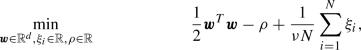(1)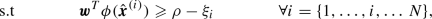(2)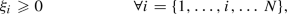(3)

where $$\nu \in (0,1)$$ is a regularization hyperparameter, $$\xi _i \in \mathbb {R}$$ are slack variables, and $$\varvec{w}$$ and $$\rho$$ are the parameters of the hyperplane in high-dimensional feature space. Schölkopf et al. (2000) show that $$\nu$$ is an upper bound on the fraction of outliers and a lower bound on the fraction of support vectors in the training set, which is known as the $$\nu$$-property. The decision function $$f_{\text {DF-P}}(\varvec{x}) = \varvec{w}^T\phi (\varvec{x})-\rho$$ is positive if a candidate point $$\varvec{x}$$ is classified to be within the training data domain and negative if not. The dual of (1)-(3) is the quadratic program: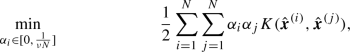(4)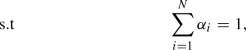(5)

where $$\alpha _i$$ are dual variables and $$K(\cdot ,\cdot )$$ is a kernel function. Often, the radial basis kernel function $$K(\varvec{x},\varvec{y}) = \exp (-\gamma \Vert \varvec{x}-\varvec{y}\Vert ^2)$$ with hyperparameter $$\gamma$$ is used since it has been shown that it is best able to model the most complex boundaries (Tax 2001). It holds that $$\alpha _i = 0$$ for training samples inside the learned boundary and $$\alpha _i > 0$$ for samples on or outside the boundaries. Samples for which $$\alpha _i > 0$$ are called support vectors. The decision function in the dual variables is given by $$f_{\text {DF-D}}(\varvec{x}) = \sum _{i \in I_{\text {sv}}} \alpha _i K(\hat{\varvec{x}}^{(i)}, \varvec{x}) -\rho$$, where $$I_{\text {sv}}$$ denotes the indexes of the support vectors in the training data (i.e., the data points with corresponding $$\alpha _i > 0$$). To obey validity limits of data-driven models in an optimization problem, the following inequality has to hold:

\begin{aligned} f_{\text {DF-D}}(\varvec{x}) \geqslant 0 . \end{aligned}
(6)

The parameter $$\nu$$ can be estimated from an outlier fraction by using the aforementioned $$\nu$$-property. This makes this method more tolerant to outliers in the training data (Pimentel et al. 2014). The hyperparameter $$\gamma$$ controls the model complexity when using the radial basis kernel. If a large $$\gamma$$ is used, all samples are mapped to a small region in the feature space and the one-class SVM cannot distinguish between the samples well. In other words, the model lacks complexity. If $$\gamma$$ is small, pairs of samples become orthogonal in the feature space. This leads to overfitting and a high number of support vectors. A common approach to identify an appropriate $$\gamma$$ is to gradually decrease $$\gamma$$ until the number of support vectors does not decrease much (e.g., Dreiseitl et al. 2010). However, automatically selecting an appropriate $$\gamma$$ is challenging (e.g.,Evangelista et al. 2007; Xiao et al. 2014a, b).

### Optimization with classifier as constraint

We consider a global optimization problem where a classifier is used to obey validity limits of a data-driven model. In most cases, the inputs of the classifier model correspond to the degrees of freedom $$\varvec{x}$$ of the optimization problem. The classifier can determine if a given $$\varvec{x}$$ is feasible or infeasible by evaluating its decision function $$f_{\text {DF-D}}(\cdot )$$. To obey validity limits, Inequality (6) has to hold. Although the decision function is an explicit function, there exist different ways to formulate it in optimization problems. These problem formulations are equivalent as they have the same solution, but they can have a large impact on the computational performance of global optimization.

In the full-space (FS) formulation, a set of nonlinear equations is provided as equality constraints while the dependent (or intermediate) variables are optimization variables. Note that there exist multiple valid FS formulations depending on the equality constraints and optimization variables provided to the solver. One representative FS formulation for optimization with one-class SVMs embedded is shown in the following: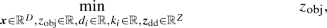(7)(8)(9)(10)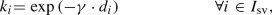(11)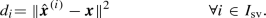(12)

Herein, Eq. (7) minimizes the objective function value $$z_{\text {obj}}$$ that is given by Eq. (8). Note that the objective depends on the variables of the data driven model $$\varvec{z}_{\text {dd}}$$ that are given by the solution of Eq. (9). The decision function of the one-class SVM is given by the inequality constraint (10) while its intermediate variables are given by the solution of Eqs. (11),(12). This FS formulation has in total $$D+2\cdot \vert I_{\text {sv}}\vert +\dim (\varvec{z}_{\text {dd}})+ 1$$ optimization variables, $$2\cdot \vert I_{\text {sv}}\vert +\dim (\varvec{z}_{\text {dd}})+ 1$$ equality constraints, and one inequality constraint.

The equality constraints of the one-class SVM can be solved explicitly for the intermediate variables. Thus, we can directly formulate a RS formulation of the optimization problem (c.f. Bongartz and Mitsos 2017):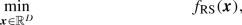(13)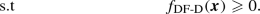(14)

Herein, $$f_{\text {RS}}(\cdot )$$ is the RS formulation of the data-driven model and objective function. Thus, Eq. (13) results from sequential substitutions of Eqs. (7)–(9). This is possible as most data-driven models such as ANNs or GPs are explicit functions (c.f. Schweidtmann and Mitsos 2019; Schweidtmann et al. 2020a) and as the objective function is a function of the degrees of freedom and the predictions of the data-driven model. Similarly, Eq. (14) results from the substitution of Eqs. (10)–(12). The RS formulation has only D optimization variables and one inequality constraint.

The convex hull of a point cloud with a finite number of points can be formulated as a set of linear inequality constraints $${X}_\text {convHull} = \{\varvec{x} \in \mathbb {R}^D~|~\varvec{A}\varvec{x}+\varvec{b} \le \varvec{0}\}$$. Assuming that the convex hull has f facets, the matrix $$\varvec{A} \in \mathbb {R}^{f\times D}$$ and the vector $$\varvec{b} \in \mathbb {R}^f$$ (Kahrs and Marquardt 2007). Thus, the FS and RS formulation of the convex hull are identical. Note that the data-driven model can still be formulated in the RS and FS formulation when using the linear convex hull constraints.

The RS formulation has three major advantages for global optimization: First, the problem formulation has a direct influence on the variables to be branched on. In the RS, the B&B solver branches only on the degrees of freedom $$\varvec{x}$$. In the FS, the B&B solver branches on the degrees of freedom and also on the intermediate variables. This is undesirable given the exponential worst-case runtime of global optimization methods. Note that this issue can also be mitigated by selective branching (Epperly and Pistikopoulos 1997). Second, the size of the subproblems that are solved during optimization is affected by the problem formulation and the method for constructing relaxations. Our previous work shows that a combination of McCormick relaxations and RS formulation can reduce the time to solve an iteration of the B&B solver significantly (Schweidtmann et al. 2020a; Bongartz 2020). Third, global optimization solvers usually require bounds on all optimization variables. Often, meaningful bounds are known for degrees of freedom but bounds on intermediate variables can be difficult to determine. Note that this problem is mitigated by some state-of-the-art solvers through automatic bound tightening techniques.

The vast majority of previous literature approaches formulate global optimization in the FS because they frequently use modeling environments such as GAMS that essentially require an equation-oriented modeling approach. Recently, Hart et al. (2017) developed the Python-based optimization tool Pyomo which allows modeling, implementation of own solvers, and provides access to multiple solvers. Pyomo allows both FS and RS and recently Hüllen et al. (2019) demonstrated RS optimization of ANNs in BARON through Pyomo. However, BARON relies on the auxiliary variable method for relaxations which results in larger subproblems (Schweidtmann et al. 2020a). Thus, a RS formulation in BARON does not take full advantage of the RS formulation. In contrast, our open-source solver MAiNGO (Bongartz et al. 2018) relies on McCormick relaxations in the space of the original variables utilizing the library MC++ (Mitsos et al. 2009; Chachuat et al. 2015). Another open-source solver that allows for McCormick relaxations is called EAGO has been released by Wilhelm and Stuber (2020).

The optimization problems in this work are implemented in MeLOn (Schweidtmann et al. 2020b) and solved by MAiNGO (Bongartz et al. 2018). For comparison, the problems are additionally exported to GAMS and solved by the commercial solver BARON (Tawarmalani and Sahinidis 2005). We provide the implementation of the one-class SVM in the open-source modeling toolbox MeLOn (Schweidtmann et al. 2020b).

Tight convex and concave relaxations are highly desirable in global optimization. Therefore, we use the tightest possible relaxations, i.e., the envelopes, of the radial basis function kernel in our solver MAiNGO. Note that we derived these envelopes in our previous work (Schweidtmann et al. 2020a) as the squared exponential covariance function in Gaussian processes is equivalent to the radial basis function kernel in SVMs.

## Illustrative case studies

As high dimensional problems are difficult to visualize, we consider eight two-dimensional data sets for illustration of the proposed method in a first step. Afterwards, we consider an engineering case study in Sect. 4. As shown in Fig. 5, the illustrative examples cover a variety of relevant scenarios. All data points are randomly generated within pre-specified bounds and perturbed by noise. Thus, the data sets do not exhibit sharp boundaries, rather they also include noisy outlier data points.

In the next step, we evaluate an adapted peaks function, $$f_{\text {Peaks}} : \mathbb {R}^2 \mapsto \mathbb {R}$$, on all data sets with $$f_{\text {Peaks}}(x_1,x_2) = 3(1-x_1)^2 \cdot \exp {(-x_1^2-(x_2+1)^2)} -10(\frac{x_1}{5}-x_1^3-x_2^5)\cdot \exp {(-x_1^2 -x_2^2)} - \frac{1}{3}\cdot \exp {(-(x_1+1)^2-x_2^2)}-1.3x_2$$. The peaks function is a standard test function in Matlab. We adapted the function slightly by adding a linear term which avoids a flat response outside the sampled domain. Then, we train individual ANNs on the eight data sets using Keras (Chollet et al. 2015). All ANNs exhibit one input layer with 2 neurons, two hidden layers with six and eight neurons, respectively, and an output layer with one neuron. The hidden layers use $$\tanh$$ activation and the output layers use linear activation. For training, the inputs are scaled onto $$[-1,1]$$ and the outputs are scaled to zero mean and unit variance. We further use a batch size of 128 and an epoch limit of 4000. Note that we omit a hyperparameter study for the ANNs because ANN training is not the focus of this work.

All optimization problems are solved one core of an Intel Xeon CPU E5-2630 v3 (2.40GHz) with 192 GB RAM and Windows Server 2016 operating system. We use a 0.001 relative and absolute optimality tolerance, a CPU time limit of 1000 s, and default settings in BARON and MAiNGO.

### Topological data analysis

The persistent diagrams of the eight input data sets are shown in Fig. 4. The Figs.  4d, e show a H$$_1$$ component with a large life span each. These correspond to the holes in the respective data sets “box w/ hole” and “circle w/ hole”. Moreover, the corresponding $$\epsilon _{\text {death}}$$ provide information about the diameter of the holes. Recall that H$$_1$$ components that are close to the diagonal have a short life span and are therefore not relevant for this analysis.

The persistent diagrams also show the existence of disjunct clusters in the data sets “two circles” and “two ovals”. In Figs. 4f, g, the H$$_0$$ components with a high $$\epsilon _{\text {death}}$$ indicate disjunct clusters and are a measure for the distance between them. Note that the $$H_0$$ components at the infinity line persist when $$\epsilon$$ goes to infinity and do not die. They correspond to the $$H_0$$ components that include all data points.

The persistent diagrams show no distinct differences between the “box”, “oval”, “box2”, and “banana” case studies. This illustrates that the method cannot distinguish between convex and nonconvex data sets in general. Therefore, the persistent diagrams cannot ensure that the convex hull is sufficient to describe the validity domain. Rather, it can only identify some cases where the convex hull is not sufficient.

### Validity domain modeling

In order to compare the one-class SVM and the convex hull, we model the input data of the eight case studies with both methods. We employ the common radial basis function kernel for the one-class SVM. We set the hyperparameter $$\nu$$ to a low value 0.03 because there are only a few outliers through noise in the data. The hyperparameter $$\gamma$$ is identified using the incremental approach described in Sect. 2.2. The selected $$\gamma$$ values are summarized in Table 1.

The learned boundaries for the case studies are depicted in Fig. 5. As expected, the convex hull does not model holes and disjunct data clusters. Instead, the convex hull overestimates the validity domain of the case studies. In contrast, the one-class SVM is able to model holes and disjunct data clusters. Furthermore, the convex hull includes all data points whereas the one-class SVM also allows for outliers in the data and excludes regions with only small data density from the validity domain (e.g., see the “box” case study). Notably, the one-class SVM is more stringent in these cases compared methods that rely on the distance to the closest training data points (Teixeira et al. 2006; Rall et al. 2019).

### Optimization results

We minimize the prediction of the eight trained ANNs subject to the convex hull or the one-class SVM as constraints. Table 2 shows the optimal solution points, $$\mathbf{x} ^*$$, and objective function values, $$f_{\text {ANN}}(\mathbf{x} ^*)$$, for the problem with convex hull and one-class SVM as constraints.

The optimal objective function values of the convex hull approach are lower than the ones with the one-class SVM for all case studies because the convex hull overestimates the validity domain. This overestimation can lead to large errors at the optimal solution points. For the “banana” case study, the optimal solution found by the convex hull approach is outside the validity domain but at the boundary of the convex hull (see Table 2). This leads to a wrongly estimated objective values by the ANN of $$-5.2$$ with an absolute error of 8.52. In contrast, the one-class SVM models the validity domain accurately and yields an optimal solution of $$-4.3$$ with an absolute error of 0.11. Also, the solution point found by the ANN model with the one-class SVM constraint is close to the reference solution where the learned peaks function is optimized subject to the SVM constraint. Similarly, the one-class SVM leads to more reliable results in the data sets “two circles”, “box w/ holes”, and “circle w/ holes”. Interestingly, the convex hull approach also leads to a substantial prediction error in the “box” case study while the one-class SVM models the validity domain accurately. This highlights the risk of using the convex hull approach in the presence of noise. Note that methods which rely on the distance to the closest training data points face similar issues.

In Table 3, we provide the CPU times for optimization with one-class SVMs embedded. Using the FS formulation, BARON and MAiNGO perform similarly and solve most problems in the a few hundred CPU seconds. The RS formulation outperforms the FS formulation on all problem instances. In BARON, the speedup factor between the RS and the FS formulation ranges from 5 to over 14. In comparison, the speedup factor between the RS and FS in MAiNGO ranges between 583 to over 3226. This is in agreement with our previous studies where the McCormick relaxations in the RS lead to smaller subproblems compared to the auxiliary variable method (see Sect. 2.3).

In Table 4, we compare the computational performance for optimization with the convex hull embedded. The CPU times with the convex hull are lower compared to the ones with one-class SVMs. MAiNGO is substantially faster than BARON when formulating the problem in the FS. On average, BARON requires about 83 s to solve the problem in the FS while MAiNGO requires only 3 s. The RS formulation again outperforms the FS formulation for all problems. However, in this case, the speedup factors are in the same order of magnitude for BARON and MAiNGO ranging between 13 and 54. It should be noted that the RS and the FS formulation of the convex hull constrains are identical. Therefore, the difference is only due to the formulation of the data-driven model in the objective function, i.e., the ANN (c.f. Schweidtmann and Mitsos 2019).

## Engineering application

We consider a sulfur recovery unit as a relevant engineering case study for our work because a large data set of industry operating data is available online for this process. The efficient recovery of sulfur in petroleum refineries from tail gas is important for environmental reasons. The sulfur recovery unit process is illustrated in Fig. 6. The process has two acid gases as inputs: the MAE gas stream is rich in hydrogen sulphide (H$$_2$$S) and comes from the gas washing plants. The SWS gas stream is rich in H$$_2$$S and ammonia and comes from a sour water stripping plant. In the sulfur recovery unit, the acid gases are burnt via partial reaction with air in a two-chamber reaction furnace. Then, the combustion product is further treated in two subsequent catalytic reactors resulting in a tail gas stream that contains residuals of H$$_2$$S and sulfur dioxide (SO$$_2$$). A detailed process description can be found in the literature (Fortuna et al. 2007).

A key issue of the sulfur recovery unit is the control of the secondary air flow to ensure optimal conditions for the total removal of the sulfur compounds in the catalytic converters. Previous works have investigated soft sensors for the tail gas concentrations of hydrogen sulphide (H$$_2$$S) and sulfur dioxide (SO$$_2$$) using ANNs and implemented those in industry for monitoring (Quek et al. 2000; Fortuna et al. 2003, 2007). In this case study, we solve an open-loop control problem to find the optimal secondary air flow rate. The objective is to minimize $$\vert c_{H_2S}-2\cdot c_{SO_2} \vert$$ such that the two reactants are in stoichiometric proportion. Similar to the previous literature by Fortuna et al. (2003, 2007), we also train two ANNs to predict the concentrations:

\begin{aligned}&c_{H_2S, k}&= f_{{ANN, H_2S}} \left( x_{1,k}, x_{1,k-5}, x_{1,k-7}, x_{1,k-9}, \ldots x_{5,k}, x_{5,k-5}, x_{5,k-7}, x_{5,k-9} \right) , \\&c_{SO_2, k}&= f_{{ANN, SO_2}} \left( x_{1,k}, x_{1,k-5}, x_{1,k-7}, x_{1,k-9}, \ldots x_{5,k}, x_{5,k-5}, x_{5,k-7}, x_{5,k-9} \right) , \end{aligned}

where $$x_{1,k}$$ is the gas flow in the MEA zone, $$x_{2,k}$$ is the air flow in the MEA zone, $$x_{3,k}$$ is the secondary air flow in the MEA zone, $$x_{4,k}$$ is the air flow in the SWS zone, $$x_{5,k}$$ is the gas flow in the SWS zone at time step k. The $$SO_2$$ ANN has one hidden layer with eight neurons and the $$H_2S$$ ANN has two hidden layers with eight neurons each. The data-driven models are trained on (scaled) industrial data collected at a plant located in Priolo, Italy available at https://www.openml.org/d/23515. The data set includes a time series with approximately 10,000 data samples and we use the first 90% of the data for training. The control variable of the NMPC is the secondary air flow $$x_{3,k}$$ while the other inputs are observable parameters. As the control is critical for process safety, the validity limits of the data-driven model should be considered.

In order to analyze the topology of the 20-dimensional input training data set of ANNs, we perform persistent homology. Due to the large number of data points, the exact computation of the persistent diagram is expensive. We apply approximate sparse filtration instead (Cavanna et al. 2015). The persistent diagram for this case study is shown in Fig. 7. The diagram shows that there exist a number of holes in the data set that persist over a long time span. Also, a separate cluster can be observed in the data. This motivates the use of one-class SVM to obey validity limits of data-driven models.

As we have no physical model of the process available, the closed-loop performance of the controller is not studied in this example. For illustration, we perform one step of an open-loop controller for the secondary air flow $$x_{3,k}$$. We select a random operating point from the historic plant data (Table 5) and let the solver determine the optimal control action. The problem is solved to global optimality within 0.33 CPU seconds and identifies a control action $$x_{3,k}=0.266$$ that results in the desired stoichiometric composition, i.e., $$\vert c_{H_2S}-2\cdot c_{SO_2} \vert =1.3\cdot 10^{-5}$$. This engineering case study also demonstrates the potential of the proposed method for NMPC. Note that deterministic global NMPC can become computationally expensive for long control horizons and higher dimensional control vectors (Chachuat et al. 2006; Doncevic et al. 2020; Kappatou et al. 2020).

## Conclusion

Safety concerns and extrapolation issues often impede industrial applications of machine learning models. We present a three-step approach to obey the validity limits of data-driven models. First, we perform a data topology analysis using persistent homology. Second, we model the validity domain of the data-driven model using either the convex hull or a one-class SVM. Third, we perform deterministic global optimization with the validity domain model as a constraint.

All used and developed methods are available open-source. Also, we currently develop a Python interface for our solver MAiNGO. Thus, all methods can be applied and further developed in academia and industry for free.

Our method has the potential to enhance safety, trust, and reliability of machine learning approaches. Moreover, we demonstrate that persistent homology is a valuable method for understanding the topology of data in high dimensional spaces. Besides industry applications, promising future work also includes the application to optimization problems occurring in molecular design where molecules are parameterized through graph neural networks (Schweidtmann et al. 2020c) or autoencoders (Jin et al. 2018). Also, time-dependent design space descriptions are desired in pharmaceutics (von Stosch et al. 2020). The proposed method can also be extended by considering and comparing other one-class classification methods. Finally, the extension of the presented method to adaptive space exploration would be promising future work.

## Availability of data and material

The (scaled) industrial data used in the engineering case study are available at https://www.openml.org/d/23515.

## References

1. Asprion N (2020) Modeling, simulation, and optimization 4.0 for a distillation column. Chem Ing Tech 92(7):879–889

2. Asprion N, Böttcher R, Pack R, Stavrou ME, Höller J, Schwientek J, Bortz M (2019) Gray-box modeling for the optimization of chemical processes. Chem Ing Tech 91(3):305–313

3. Bhosekar A, Ierapetritou M (2018) Advances in surrogate based modeling, feasibility analysis, and optimization: a review. Comput Chem Eng 108:250–267

4. Binchi J, Merelli E, Rucco M, Petri G, Vaccarino F (2014) jholes: a tool for understanding biological complex networks via clique weight rank persistent homology. Electron Notes Theor Comput Sci 306:5–18

5. Bongartz D (2020) Deterministic global flowsheet optimization for the design of energy conversion processes. Ph.D. thesis, RWTH Aachen University

6. Bongartz D, Mitsos A (2017) Deterministic global optimization of process flowsheets in a reduced space using McCormick relaxations. J Global Optim 20(9):419

7. Bongartz D, Najman J, Sass S, Mitsos A (2018) MAiNGO: McCormick-based algorithm for mixed integer nonlinear global optimization. Technical report, Process Systems Engineering (AVTSVT), RWTH Aachen University. http://permalink.avt.rwth-aachen.de/?id=729717

8. Boukouvala F, Ierapetritou MG (2012) Feasibility analysis of black-box processes using an adaptive sampling kriging-based method. Comput Chem Eng 36:358–368

9. Cavanna NJ, Jahanseir M, Sheehy DR (2015) A geometric perspective on sparse filtrations. arXiv:1506.03797

10. Chachuat B, Singer AB, Barton PI (2006) Global methods for dynamic optimization and mixed-integer dynamic optimization. Ind Eng Chem Res 45(25):8373–8392

11. Chachuat B, Houska B, Paulen R, Peric N, Rajyaguru J, Villanueva ME (2015) Set-theoretic approaches in analysis, estimation and control of nonlinear systems. IFAC-PapersOnLine 48(8):981–995. https://doi.org/10.1016/j.ifacol.2015.09.097

12. Chambers EW, Letscher D (2018) Persistent homology over directed acyclic graphs. In: Research in computational topology. Springer, pp 11–32

13. Chandola V, Banerjee A, Kumar V (2009) Anomaly detection: a survey. ACM Comput Sur (CSUR) 41(3):1–58

14. Charnes A, Cooper WW (1959) Chance-constrained programming. Manage Sci 6(1):73–79

15. Chazal F, Michel B (2017) An introduction to topological data analysis: fundamental and practical aspects for data scientists. arXiv:1710.04019

16. Chen Q, Paulavičius R, Adjiman CS, García-Muñoz S (2018) An optimization framework to combine operable space maximization with design of experiments. AIChE J 64(11):3944–3957

17. Chollet F et al (2015) Keras. https://keras.io. Accessed May 2020

18. Chung MK, Hanson JL, Ye J, Davidson RJ, Pollak SD (2015) Persistent homology in sparse regression and its application to brain morphometry. IEEE Trans Med Imaging 34(9):1928–1939

19. Cortes C, Vapnik V (1995) Support-vector networks. Mach Learn 20(3):273–297

20. Courrieu P (1994) Three algorithms for estimating the domain of validity of feedforward neural networks. Neural Netw 7(1):169–174

21. Ding X, Li Y, Belatreche A, Maguire LP (2014) An experimental evaluation of novelty detection methods. Neurocomputing 135:313–327

22. Doncevic DT, Schweidtmann AM, Vaupel Y, Schäfer P, Caspari A, Mitsos A (2020) Deterministic global nonlinear model predictive control with recurrent neural networks embedded. In: IFAC conference proceedings (in press)

23. Dreiseitl S, Osl M, Scheibböck C, Binder M (2010) Outlier detection with one-class svms: an application to melanoma prognosis. In: AMIA annual symposium proceedings, vol 2010. American Medical Informatics Association, p 172

24. Epperly TGW, Pistikopoulos EN (1997) A reduced space branch and bound algorithm for global optimization. J Global Optim 11(3):287–311

25. Evangelista PF, Embrechts MJ, Szymanski BK (2007) Some properties of the Gaussian kernel for one class learning. In: International conference on artificial neural networks. Springer, pp 269–278

26. Fortuna L, Rizzo A, Sinatra M, Xibilia M (2003) Soft analyzers for a sulfur recovery unit. Control Eng Pract 11(12):1491–1500

27. Fortuna L, Graziani S, Rizzo A, Xibilia MG (2007) Soft sensors for monitoring and control of industrial processes. Springer

28. Glassey J, Von Stosch M (2018) Hybrid modeling in process industries. CRC Press

29. Hart WE, Laird CD, Watson JP, Woodruff DL, Hackebeil GA, Nicholson BL, Siirola JD (2017) Pyomo-optimization modeling in python, vol 67. Springer

30. Hiraoka Y, Nakamura T, Hirata A, Escolar EG, Matsue K, Nishiura Y (2016) Hierarchical structures of amorphous solids characterized by persistent homology. Proc Natl Acad Sci 113(26):7035–7040

31. Hüllen G, Zhai J, Kim SH, Sinha A, Realff MJ, Boukouvala F (2019) Managing uncertainty in data-driven simulation-based optimization. Comput Chem Eng. https://doi.org/10.1016/j.compchemeng.2019.106519

32. Jin W, Barzilay R, Jaakkola T (2018) Junction tree variational autoencoder for molecular graph generation. arXiv:1802.04364

33. Kahrs O, Marquardt W (2007) The validity domain of hybrid models and its application in process optimization. Chem Eng Process 46(11):1054–1066

34. Kahrs O, Marquardt W (2008) Incremental identification of hybrid process models. Comput Chem Eng 32(4–5):694–705

35. Kappatou CD, Bongartz D, Najman J, Sass S, Mitsos A (2020) Global dynamic optimization with hammerstein-wiener models embedded. http://www.optimization-online.org/DB_HTML/2020/09/8018.html

36. Khan SS, Madden MG (2009) A survey of recent trends in one class classification. In: Irish conference on artificial intelligence and cognitive science. Springer, pp 188–197

37. Khan SS, Madden MG (2014) One-class classification: taxonomy of study and review of techniques. Knowl Eng Rev 29(3):345–374

38. Kimura Y, Imai K (2017) Quantification of LSS using the persistent homology in the SDSS fields. Adv Space Res 60(3):722–736

39. Knudde N, Couckuyt I, Shintani K, Dhaene T (2019) Active learning for feasible region discovery. In: 2019 18th IEEE international conference on machine learning and applications (ICMLA). IEEE, pp 567–572

40. Kumar JN, Li Q, Tang KY, Buonassisi T, Gonzalez-Oyarce AL, Ye J (2019) Machine learning enables polymer cloud-point engineering via inverse design. NPJ Comput Mater 5(1):1–6

41. Larson BJ, Mattson CA (2012) Design space exploration for quantifying a system model’s feasible domain. ASME J Mech Des 134(4):041010. https://doi.org/10.1115/1.4005861

42. Leonard J, Kramer MA, Ungar L (1992) A neural network architecture that computes its own reliability. Comput Chem Eng 16(9):819–835

43. Letscher H, Edelsbrunner D, Zomorodian A (2002) Topological persistence and simplification. Discrete Comput Geom 28:511–533

44. Malak RJ Jr, Paredis CJJ (2010) Using support vector machines to formalize the valid input domain of predictive models in systems design problems. ASME J Mech Des 132(10):101001. https://doi.org/10.1115/1.4002151

45. McBride K, Sundmacher K (2019) Overview of surrogate modeling in chemical process engineering. Chem Ing Tech 91(3):228–239. https://doi.org/10.1002/cite.201800091

46. Mistry M, Letsios D, Krennrich G, Lee RM, Misener R (2018) Mixed-integer convex nonlinear optimization with gradient-boosted trees embedded. arXiv:1803.00952

47. Mitsos A, Chachuat B, Barton PI (2009) McCormick-based relaxations of algorithms. SIAM J Optim 20(2):573–601. https://doi.org/10.1137/080717341

48. Mogk G, Mrziglod T, Schuppert A (2002) Application of hybrid models in chemical industry. In: Computer aided chemical engineering, vol 10. Elsevier, pp 931–936

49. Otter N, Porter MA, Tillmann U, Grindrod P, Harrington HA (2017) A roadmap for the computation of persistent homology. EPJ Data Sci 6(1):17

50. Papadopoulos G, Edwards PJ, Murray AF (2001) Confidence estimation methods for neural networks: a practical comparison. IEEE Trans Neural Netw 12(6):1278–1287

51. Patania A, Vaccarino F, Petri G (2017) Topological analysis of data. EPJ Data Sci 6:1–6

52. Pedregosa F, Varoquaux G, Gramfort A, Michel V, Thirion B, Grisel O, Blondel M, Prettenhofer P, Weiss R, Dubourg V et al (2011) Scikit-learn: machine learning in python. J Mach Learn Res 12:2825–2830

53. Pimentel MA, Clifton DA, Clifton L, Tarassenko L (2014) A review of novelty detection. Sig Process 99:215–249

54. Pinto J, de Azevedo CR, Oliveira R, von Stosch M (2019) A bootstrap-aggregated hybrid semi-parametric modeling framework for bioprocess development. Bioprocess Biosyst Eng 42(11):1853–1865

55. Quaglio M, Fraga ES, Cao E, Gavriilidis A, Galvanin F (2018) A model-based data mining approach for determining the domain of validity of approximated models. Chemometr Intell Lab Syst 172:58–67

56. Quek C, Balasubramanian R, Rangaiah G (2000) Consider using soft analyzers to improve SRU control. Hydrocarbon processing 79(1):101–106

57. Rall D, Menne D, Schweidtmann AM, Kamp J, von Kolzenberg L, Mitsos A, Wessling M (2019) Rational design of ion separation membranes. J Membr Sci 569:209–219

58. Roach E, Parker RR, Malak RJ Jr (2011) An improved support vector domain description method for modeling valid search domains in engineering design problems. Int Des Eng Tech Conf Comput Inf Eng Conf 54822:741–751

59. Saadatfar M, Takeuchi H, Robins V, Francois N, Hiraoka Y (2017) Pore configuration landscape of granular crystallization. Nat Commun 8(1):1–11

60. Schölkopf B (2001) The kernel trick for distances. In: Advances in neural information processing systems, pp 301–307

61. Schölkopf B, Williamson RC, Smola AJ, Shawe-Taylor J, Platt JC (2000) Support vector method for novelty detection. In: Advances in neural information processing systems, pp 582–588

62. Schweidtmann AM, Bongartz D, Grothe D, Kerkenhoff T, Lin X, Najman J, Mitsos A (2020a) Global optimization of Gaussian processes. arXiv:2005.10902

63. Schweidtmann AM, Netze L, Mitsos A (2020b) Melon: Machine learning models for optimization. https://git.rwth-aachen.de/avt.svt/public/MeLOn/

64. Schweidtmann AM, Rittig JG, König A, Grohe M, Mitsos A, Dahmen M (2020c) Graph neural networks for prediction of fuel ignition quality. ChemRxiv preprint ChemRxiv:12280325

65. Schweidtmann AM, Mitsos A (2019) Deterministic global optimization with artificial neural networks embedded. J Optim Theory Appl 180(3):925–948

66. Shahriari B, Swersky K, Wang Z, Adams RP, de Freitas N (2016) Taking the human out of the loop: a review of bayesian optimization. Proc IEEE 104(1):148–175. https://doi.org/10.1109/JPROC.2015.2494218

67. Simutis R, Havlik I, Schneider F, Dors M, Lübbert A (1995) Artificial neural networks of improved reliability for industrial process supervision. IFAC Proc Vol 28(3):59–65

68. Smith AD, Dlotko P, Zavala VM (2020) Topological data analysis: concepts, computation, and applications in chemical engineering. arXiv:2006.03173

69. Smola AJ, Schölkopf B (2004) A tutorial on support vector regression. Stat Comput 14(3):199–222

70. Tawarmalani M, Sahinidis NV (2005) A polyhedral branch-and-cut approach to global optimization. Math Program 103(2):225–249. https://doi.org/10.1007/s10107-005-0581-8

71. Tax DMJ (2001) One-class classification: Concept learning in the absence of counter-examples. Ph.D. thesis, Delft University of Technology

72. Tax DM, Duin RP (1999) Data domain description using support vectors. ESANN 99:251–256

73. Teixeira AP, Clemente JJ, Cunha AE, Carrondo MJ, Oliveira R (2006) Bioprocess iterative batch-to-batch optimization based on hybrid parametric/nonparametric models. Biotechnol Prog 22(1):247–258

74. Tralie C, Saul N, Bar-On R (2018) Ripser.py: a lean persistent homology library for python. J Open Source Softw 3(29):925

75. Venkatasubramanian V (2019) The promise of artificial intelligence in chemical engineering: is it here, finally. AIChE J 65(2):466–78

76. Virtanen P, Gommers R, Oliphant TE, Haberland M, Reddy T, Cournapeau D, Burovski E, Peterson P, Weckesser W, Bright J et al (2020) Scipy 1.0: fundamental algorithms for scientific computing in python. Nat Methods 17(3):261–272

77. Von Stosch M, Oliveira R, Peres J, de Azevedo SF (2014) Hybrid semi-parametric modeling in process systems engineering: past, present and future. Comput Chem Eng 60:86–101. https://doi.org/10.1016/j.compchemeng.2013.08.008

78. von Stosch M, Schenkendorf R, Geldhof G, Varsakelis C, Mariti M, Dessoy S, Vandercammen A, Pysik A, Sanders M (2020) Working within the design space: do our static process characterization methods suffice? Pharmaceutics 12(6):562

79. Wasserman L (2018) Topological data analysis. Ann Rev Stat Appl 5:501–532

80. Wilhelm ME, Stuber MD (2020) EAGO.jl: easy advanced global optimization in Julia. Optim Methods Softw. https://doi.org/10.1080/10556788.2020.1786566

81. Xia K (2018) Persistent homology analysis of ion aggregations and hydrogen-bonding networks. Phys Chem Chem Phys 20(19):13448–13460

82. Xia K, Anand DV, Shikhar S, Mu Y (2019) Persistent homology analysis of osmolyte molecular aggregation and their hydrogen-bonding networks. Phys Chem Chem Phys 21(37):21038–21048

83. Xiao Y, Wang H, Xu W (2014a) Parameter selection of Gaussian kernel for one-class svm. IEEE Trans Cybern 45(5):941–953

84. Xiao Y, Wang H, Zhang L, Xu W (2014b) Two methods of selecting Gaussian kernel parameters for one-class svm and their application to fault detection. Knowl-Based Syst 59:75–84

85. Zhang Q, Grossmann IE, Sundaramoorthy A, Pinto JM (2016) Data-driven construction of convex region surrogate models. Optim Eng 17(2):289–332. https://doi.org/10.1007/s11081-015-9288-8

86. Zomorodian A, Carlsson G (2005) Computing persistent homology. Discrete Comput Geom 33(2):249–274

## Acknowledgements

We are grateful to Benoît Chachuat for providing MC++ under Eclipse Public License. We also thank Dominik Bongartz and Jaromił Najman for their work on MAiNGO.

## Funding

Open Access funding enabled and organized by Projekt DEAL. This work was supported by the Deutsche Forschungsgemeinschaft (DFG, German Research Foundation) under Germany’s Excellence Strategy - Cluster of Excellence 2186 “The Fuel Science Center”.

## Author information

Authors

### Contributions

AMS and JMW designed the research concept. AMS wrote the manuscript. JMW run the persistent homology analyzed the persistent plots, and wrote the corresponding method and result sections. AMS, CW, and LN run the optimization. AMS, LN, and CW implemented the model in the MeLOn tool. AM is principal investigator who guided the effort and edited the manuscript.

### Corresponding author

Correspondence to Artur M. Schweidtmann.

## Ethics declarations

### Conflicts of interest

The authors declare that they have no conflict of interest.

### Code availability

The method is ready-to-use and available open-source as part of our “MeLOn - Machine Learning Models for Optimization” toolbox under the Eclipse public license (https://git.rwth-aachen.de/avt.svt/public/MeLOn).